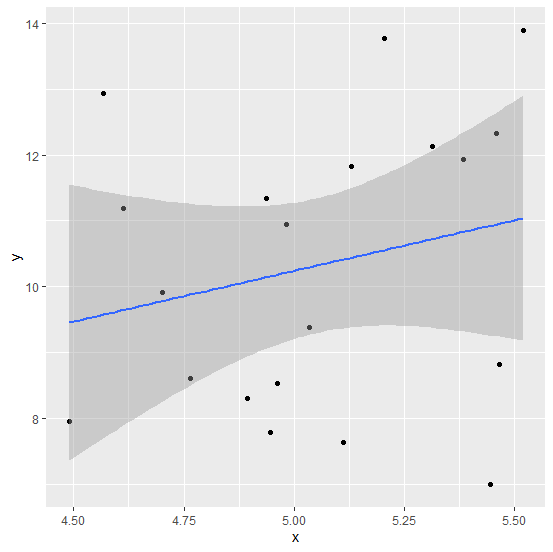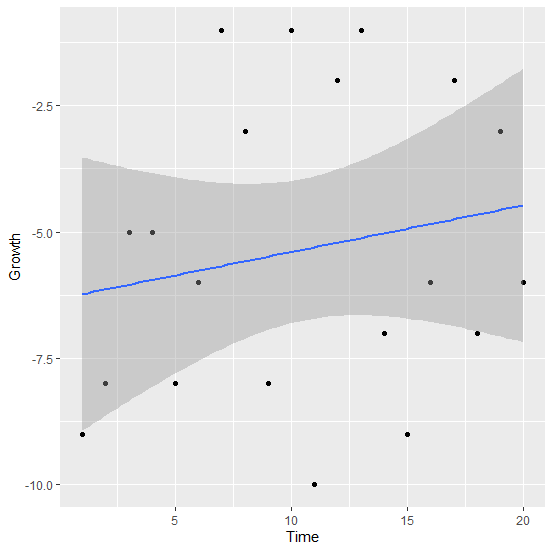# How to create regression model line in a scatterplot created by using ggplot2 in R?

To add a regression model line in a scatterplot created by using ggplot2, we need to use geom_smooth function to define the line for linear model. For example, if we have a data frame df that contains independent variable x and the dependent variable y then the regression line can be created by using the code −

ggplot(df,aes(x,y))+geom_point()+geom_smooth(method="lm")

## Example

Consider the below data frame −

Live Demo

set.seed(133)
x<-rnorm(20,5,0.375)
y<-rnorm(20,10,2)
df<-data.frame(x,y)
df

## Output

      x       y
1 5.033896 9.383918
2 5.130221 11.829104
3 4.702206 9.917457
4 5.110479 7.629133
5 5.464191 8.825002
6 4.982272 10.948461
7 5.382662 11.926883
8 4.961799 8.531400
9 4.936903 11.334979
10 4.489832 7.950781
11 5.518205 13.898027
12 5.458050 12.322746
13 4.567242 12.939460
14 5.312169 12.133224
15 4.764677 8.614166
16 5.444759 6.995418
17 4.946610 7.791502
18 5.204590 13.769303
19 4.613702 11.185987
20 4.894561 8.297381

Loading ggplot2 package and creating regression model line in the scatterplot −

## Example

library(ggplot2)
ggplot(df,aes(x,y))+geom_point()+geom_smooth(method="lm")
geom_smooth() using formula 'y ~ x'

## OutputLet’s have a look at another example −

## Example

Live Demo

Time<-1:20
Growth<-sample(-10:-1,20,replace=TRUE)
df_growthstudy<-data.frame(Time,Growth)
df_growthstudy

## Output

 Time Growth
1  1    -9
2  2    -8
3  3    -5
4  4    -5
5  5    -8
6  6    -6
7  7    -1
8  8    -3
9  9    -8
10 10   -1
11 11   -10
12 12   -2
13 13   -1
14 14   -7
15 15   -9
16 16   -6
17 17   -2
18 18   -7
19 19   -3
20 20   -6

## Example

ggplot(df_growthstudy,aes(Time,Growth))+geom_point()+geom_smooth(method="lm")
geom_smooth() using formula 'y ~ x'

## Output